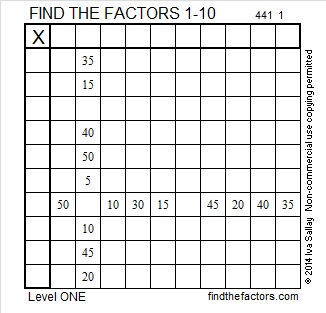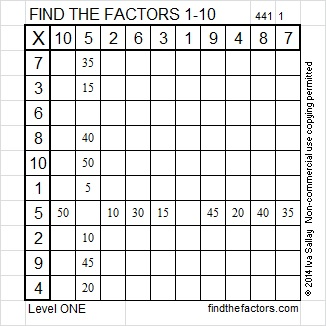# 259 and Level 1

• 259 is a composite number.
• Prime factorization: 259 = 7 x 37
• The exponents in the prime factorization are 1 and 1. Adding one to each and multiplying we get (1 + 1)(1 + 1) = 2 x 2 = 4. Therefore 259 has 4 factors.
• Factors of 259: 1, 7, 37, 259
• Factor pairs: 259 = 1 x 259 or 7 x 37
• 259 has no square factors that allow its square root to be simplified. √259 ≈ 16.093Here’s a level 1 Find the Factors puzzle:Print the puzzles or type the factors on this excel file: 10 Factors 2014-10-13This site uses Akismet to reduce spam. Learn how your comment data is processed.